## 【java算法】赫夫曼树（Huffman）的构建和应用（编码、译码）

2021/1/13 20:41:01 文章标签: 测试文章如有侵权请发送至邮箱809451989@qq.com投诉后文章立即删除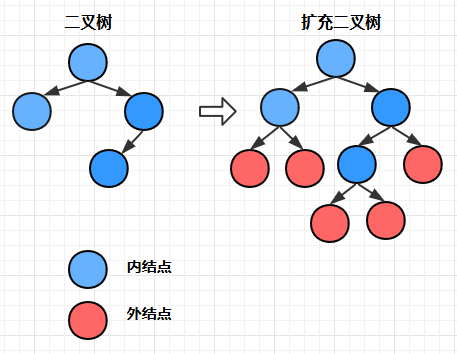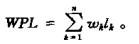（注意只是外结点！）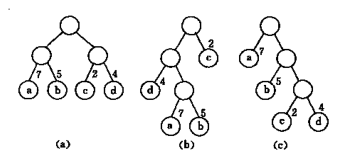c中WPL最小， 可以验证， 它就是赫夫曼树， 而a和b都不是赫夫曼树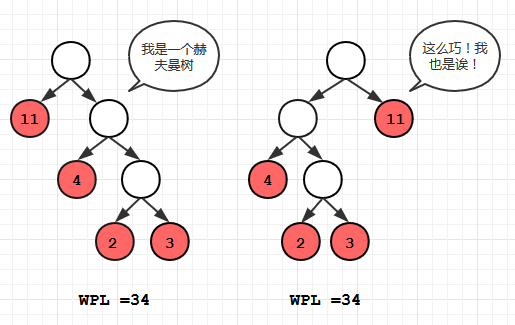1. 根据给定的n个权值{w1, w2, w3 … wn }构成n棵二叉树的集合， 每棵二叉树都只包含一个结点
2. 在上面的二叉树中选出两颗根结点权值最小的树， 同时另外取一个新的结点作为这两颗树的根结点， 设新节点的权值为两颗权值最小的树的权值和， 将得到的这颗树也加入到树的集合中
3. 在2操作后， 从集合中删除权值最小的那两颗树
4. 重复2和3，直到集合中的树只剩下一棵为止， 剩下的这颗树就是我们要求得的赫夫曼树。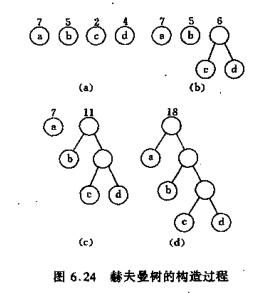（注意a和b的分界线在4和7中间，图中画的不是很清晰）

Node类的设计

``````/**
* @Author: HuWan Peng
* @Date Created in 23:21 2018/1/14
*/
public class Node {
char  data; // 数据
int weight;  // 权值
int left, right, parent; // 三条链接
public Node (char data, int weight) {
this.data = data;
this.weight = weight;
}//需要获取资料的朋友请加Q君样：290194256*
public Node (int weight) {
this.weight = weight;
}
}
``````

buildTree方法的设计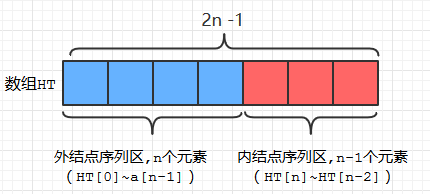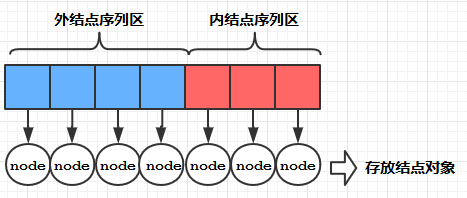1. 初始化HT中的结点对象，此时各个结点对象的weight都被置为0
2. 将输入的nodes数组中的各结点对象的权值赋给HT~ HT[n-1]， 如上图所示
3.通过循环， 依次计算各个内结点的权值，同时建立该内结点和作为它左右孩子的两个外结点的链接关系。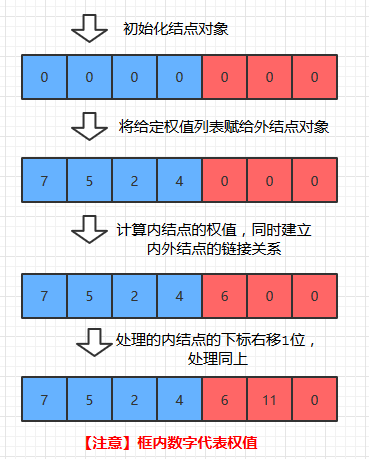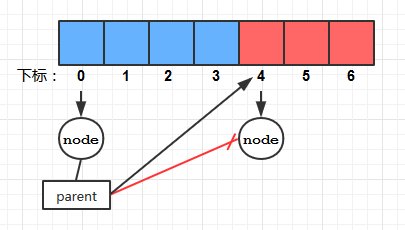（select方法尚未给出）

``````  /**
* @description: 构建赫夫曼树
*/
public  Node[] buildTree (Node [] nodes) {
int s1, s2,p;
int n = nodes.length; // 外结点的数量
int m = 2*n - 1; // 内结点 + 外结点的总数量
Node [] HT = new Node [m]; // 存储结点对象的HT数组
for (int i=0;i<m;i++) HT[i] = new Node(0); // 初始化HT数组元素
for (int i=0;i<n;i++) {
HT[i].data   = nodes[i].data;
HT[i].weight = nodes[i].weight; //将给定的权值列表赋给外结点对象
}//需要获取资料的朋友请加Q君样：290194256*
for (int i=n;i<m;i++) {
s1 = select(HT,i,0); // 取得HT数组中权值最小的结点对象的下标
s2 = select(HT,i,1); // 取得HT数组中权值次小的结点对象的下标
HT[i].left  = s1; // 建立链接
HT[i].right = s2;
HT[s1].parent = i;
HT[s2].parent = i;
HT[i].weight = HT[s1].weight + HT[s2].weight;// 计算当前外结点的权值
selectStart+=2; // 这个变量表示之前“被删除”的最小结点的数量和
}
return HT; // 将处理后的HT数组返回
}
``````

buildTree方法的用例：

``````  /**
* @description: buildTree方法的用例
*/
public static void main (String [] args) {
Node [] nodes = new Node;
nodes = new Node('a',7);
nodes = new Node('b',5);
nodes = new Node('c',2);
nodes = new Node('d',4);
HuffmanTree ht = new HuffmanTree();
Node [] n = ht.buildTree(nodes);  // n是构建完毕的赫夫曼树
}
}
``````

elect方法的设计

buildTree方法的实现依赖于select方法：

``````private  int select (Node[] HT,int range, int rank)
``````

``````s1 = select(HT,i,0); // 取得HT数组中权值最小的结点对象的下标
s2 = select(HT,i,1); // 取得HT数组中权值次小的结点对象的下标
``````

1. 求给定权值排名的结点，可以先对数组进行从小到大的快速排序， 然后就可以取得给定排名的结点对象了， 但是如果直接对输入的HT数组进行排序的话， 会改变HT数组元素的排列顺序， 这将不利于我们下面要介绍的赫夫曼编码的方法的实现。 所以这里我们先将HT数组拷贝到一个辅助数组copyNodes中， 对copyNodes进行快排，并取得给定权值排名的结点对象。然后通过遍历HT数组，比较得到该结点对象在HT中的下标
1. 在上面我们提到过， 在构建一颗新二叉树后， 要把原来的两颗权值最小的树从集合中 ”删除“，这里我们通过类内的selectStart实例变量实现， selectStart初始值为0， 每次构建一棵新二叉树后都通过 selectStart+=2; 增加它的值。（见上文buildTree代码） 这样， 在select方法中就可以通过copyNodes[selectStart + rank]，去取得 “删除” 后权值排名为rank的结点对象了。
1. 引入range这一参数是为了排除那些weight仍为0，即尚未使用到的内结点， 防止排序后取到它们。注意， 随着循环中 i 的增长， range也是不断增长的：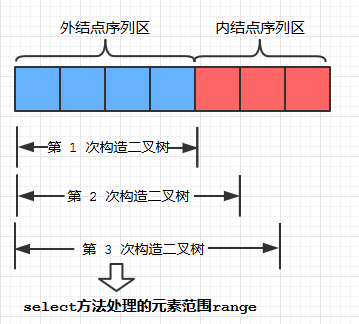（QuickSort的代码文末将给出）

``````  /**
* @description: 返回权值排名为rank的结点对象在HT中的下标（按权值从小到大排）
*/
private  int select (Node[] HT,int range, int rank) {
Node [] copyNodes = Arrays.copyOf(HT, range);// 将HT~HT[range]拷贝到copyNodes中
QuickSort.sort(copyNodes); // 对copyNodes进行从小到大的快速排序
Node target = copyNodes[rank + selectStart]; // 取得“删除”后权值排名为rank的结点对象
for (int j=0;j<HT.length;j++) {
if (target == HT[j]) return j; // 返回该结点对象在数组HT中的下标
}//需要获取资料的朋友请加Q君样：290194256*
return -1;
}
``````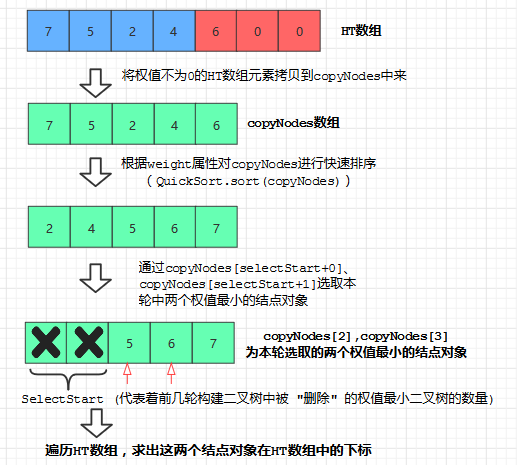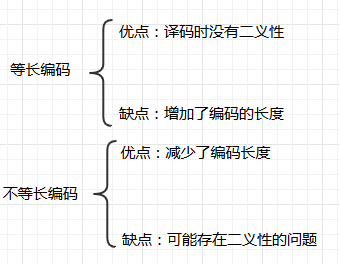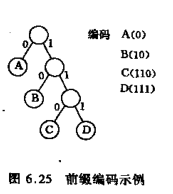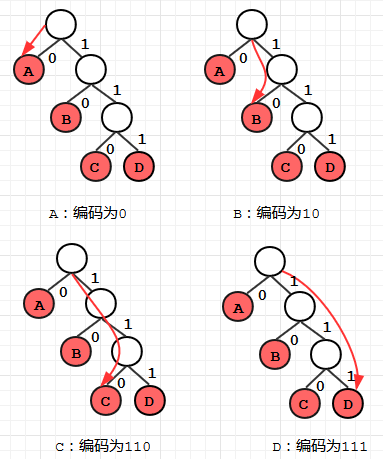1. 我们编写一个HuffmanCode内部类用于存放字符（data实例变量）和它对应的二进制字符串（bit实例变量）

2. 要求所有字符对应的编码时，如果采用从根结点下行到叶结点的思路处理，逻辑会相对复杂一些， 所以我们用逆向的方式获取： 按照从叶子结点到根结点的路径累加二进制字符串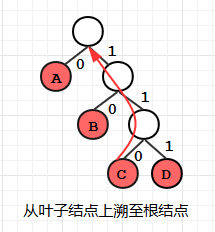1. 因为 2 的原因， 累加二进制字符串的时候也必须反向累加，例如写成bit= “0” + bit; 而不是写成bit= bit+ “0”;

2. 上溯时候要做的工作是： 判断当前经过的是 0 还是 1， 判断的方法如下图所示：
假设P是X的父节点：

X在HT中的下标，则说明X是P的右分支，说明经过的是 1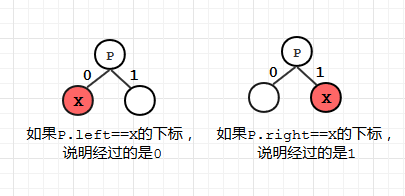``````import java.util.Arrays;
/**
* @Author: HuWan Peng
* @Date Created in 22:54 2018/1/14
*/
public class HuffmanTree {
private class HuffmanCode {
char data; // 存放字符，例如 'C'
String bit; // 存放编码后的字符串, 例如"111"
public HuffmanCode (char data, String bit) {
this.data = data;
this.bit  = bit;
}//需要获取资料的朋友请加Q君样：290194256*
}

/**
* @description: 构建赫夫曼树
*/
public  Node[] buildTree (Node [] nodes) {
// 具体代码见上文
}

/**
* @description: 进行赫夫曼编码
*/
public  HuffmanCode [] encode(Node [] nodes) {
Node [] HT = buildTree(nodes); // 根据输入的nodes数组构造赫夫曼树
int n = nodes.length;
HuffmanCode [] HC = new HuffmanCode [n];
String bit;
for (int i=0;i<n;i++) { // 遍历各个叶子结点
bit = "";
for (int c=i,f=HT[i].parent;f!=0;c=f,f=HT[f].parent) { // 从叶子结点上溯到根结点
if(HT[f].left == c) bit= "0" + bit; // 反向编码
else                bit= "1" + bit;
}
HC[i] = new HuffmanCode(HT[i].data,bit); // 将字符和对应的编码存储起来
}
return HC;
}//需要获取资料的朋友请加Q君样：290194256*

/**
* @description: encode方法的用例
*/
public static void main (String [] args) {
Node [] nodes = new Node;
nodes = new Node('A',7);
nodes = new Node('B',5);
nodes = new Node('C',2);
nodes = new Node('D',4);
HuffmanTree ht = new HuffmanTree();
HuffmanCode[] hc = ht.encode(nodes);
// 对A，B，C，D进行编码
for (int i=0;i<hc.length;i++) { // 将赫夫曼编码打印出来
System.out.println(hc[i].data + ":" +hc[i].bit);
}
}
}
``````

``````A:0
B:10
C:110
D:111
``````

``````import java.util.Arrays;

/**
* @Author: HuWan Peng
* @Date Created in 22:54 2018/1/14
*/
public class HuffmanTree {
int selectStart = 0;
private class HuffmanCode {
char data; // 存放字符，例如 'C'
String bit; // 存放编码后的字符串, 例如"111"
public HuffmanCode (char data, String bit) {
this.data = data;
this.bit  = bit;
}//需要获取资料的朋友请加Q君样：290194256*
}

/**
* @description: 构建赫夫曼树
*/
public  Node[] buildTree (Node [] nodes) {
// 代码见上文
}

/**
* @description: 进行赫夫曼译码
*/
public String decode (Node [] nodes, String code) {
String str="";
Node [] HT = buildTree(nodes);
int n =HT.length -1;
for (int i=0;i<code.length();i++) {
char c = code.charAt(i);
if(c == '1') {
n = HT[n].right;
}
else {
n = HT[n].left;
}//需要获取资料的朋友请加Q君样：290194256*
if(HT[n].left == 0) {
str+= HT[n].data;
n =HT.length -1;
}
}
return str;
}
/**
* @description: decode方法的用例
*/
public static void main (String [] args) {
Node [] nodes = new Node;
nodes = new Node('A',7);
nodes = new Node('B',5);
nodes = new Node('C',2);
nodes = new Node('D',4);
HuffmanTree ht = new HuffmanTree();
// 对 010110111 进行译码
System.out.println(ht.decode(nodes,"010110111"));
}
}
``````

``````ABCD
``````

1.HuffmanTree.java
2.Node.java
3.QuickSort.java

Node.java

``````/**
* @Author: HuWan Peng
* @Date Created in 23:21 2018/1/14
*/
public class Node {
char  data;
int weight;
int left, right, parent;
public Node (char data, int weight) {
this.data = data;
this.weight = weight;
}//需要获取资料的朋友请加Q君样：290194256*
public Node (int weight) {
this.weight = weight;
}
}
``````

HuffmanTree.java

``````import java.util.Arrays;
/**
* @Author: HuWan Peng
* @Date Created in 22:54 2018/1/14
*/
public class HuffmanTree {
int selectStart = 0;
private class HuffmanCode {
char data; // 存放字符，例如 'C'
String bit; // 存放编码后的字符串, 例如"111"
public HuffmanCode (char data, String bit) {
this.data = data;
this.bit  = bit;
}
}
/**
* @description: 返回权值排名为rank的结点对象在nodes中的下标（按权值从小到大排）
*/
private  int select (Node[] HT,int range, int rank) {
Node [] copyNodes = Arrays.copyOf(HT, range);// 将HT~HT[range]拷贝到copyNodes中
QuickSort.sort(copyNodes); // 对copyNodes进行从小到大的快速排序
Node target = copyNodes[rank + selectStart]; // 取得“删除”后权值排名为rank的结点对象
for (int j=0;j<HT.length;j++) {
if (target == HT[j]) return j; // 返回该结点对象在数组HT中的下标
}
return -1;
}//需要获取资料的朋友请加Q君样：290194256*

/**
* @description: 构建赫夫曼树
*/
public  Node[] buildTree (Node [] nodes) {
int s1, s2,p;
int n = nodes.length; // 外结点的数量
int m = 2*n - 1; // 内结点 + 外结点的总数量
Node [] HT = new Node [m]; // 存储结点对象的HT数组
for (int i=0;i<m;i++) HT[i] = new Node(0); // 初始化HT数组元素
for (int i=0;i<n;i++) {
HT[i].data   = nodes[i].data;
HT[i].weight = nodes[i].weight; //将给定的权值列表赋给外结点对象
}
for (int i=n;i<m;i++) {
s1 = select(HT,i,0); // 取得HT数组中权值最小的结点对象的下标
s2 = select(HT,i,1); // 取得HT数组中权值次小的结点对象的下标
HT[i].left  = s1; // 建立链接
HT[i].right = s2;
HT[s1].parent = i;
HT[s2].parent = i;
HT[i].weight = HT[s1].weight + HT[s2].weight;// 计算当前外结点的权值
selectStart+=2; // 这个变量表示之前“被删除”的最小结点的数量和
}
return HT; // 将处理后的HT数组返回
}

/**
* @description: 进行赫夫曼编码
*/
public  HuffmanCode [] encode(Node [] nodes) {
Node [] HT = buildTree(nodes); // 根据输入的nodes数组构造赫夫曼树
int n = nodes.length;
HuffmanCode [] HC = new HuffmanCode [n];
String bit;
for (int i=0;i<n;i++) { // 遍历各个叶子结点
bit = "";
for (int c=i,f=HT[i].parent;f!=0;c=f,f=HT[f].parent) { // 从叶子结点上溯到根结点
if(HT[f].left == c) bit= "0" + bit; // 反向编码
else                bit= "1" + bit;
}
HC[i] = new HuffmanCode(HT[i].data,bit); // 将字符和对应的编码存储起来
}
return HC;
}

/**
* @description: 进行赫夫曼译码
*/
public String decode (Node [] nodes, String code) {
String str="";
Node [] HT = buildTree(nodes);
int n =HT.length -1;
for (int i=0;i<code.length();i++) {
char c = code.charAt(i);
if(c == '1') {
n = HT[n].right;
}
else {
n = HT[n].left;
}
if(HT[n].left == 0) {
str+= HT[n].data;
n =HT.length -1;
}
}
return str;
}
/**
* @description: buildTree方法的用例
*/
public static void main (String [] args) {
Node [] nodes = new Node;
nodes = new Node('A',7);
nodes = new Node('B',5);
nodes = new Node('C',2);
nodes = new Node('D',4);
HuffmanTree ht = new HuffmanTree();
System.out.println(ht.decode(nodes,"010110111"));
}
}
``````

QuickSort.java

``````/**
* @Author: HuWan Peng
* @Date Created in 22:56 2018/1/14
*/
public class QuickSort {
/**
* @description: 交换两个数组元素
*/
private static void exchange(Node [] a , int i, int j) {
Node temp = a[i];
a[i] = a[j];
a[j] = temp;
}//需要获取资料的朋友请加Q君样：290194256*

/**
* @description: 切分函数
*/
private static int partition (Node [] a, int low, int high) {
int i = low, j = high+1;      // i, j为左右扫描指针
int pivotkey = a[low].weight;  // pivotkey 为选取的基准元素（头元素）
while(true) {
while (a[--j].weight>pivotkey) {   if(j == low) break; }  // 右游标左移
while(a[++i].weight<pivotkey) {   if(i == high) break;  }  // 左游标右移
if(i>=j) break;    // 左右游标相遇时候停止， 所以跳出外部while循环
else exchange(a,i, j) ;  // 左右游标未相遇时停止, 交换各自所指元素，循环继续
}
exchange(a, low, j); // 基准元素和游标相遇时所指元素交换，为最后一次交换
return j;  // 一趟排序完成， 返回基准元素位置
}
/**
* @description: 根据给定的权值对数组进行排序
*/
private static void sort (Node [] a, int low, int high) {
if(high<= low) { return; } // 当high == low, 此时已是单元素子数组，自然有序， 故终止递归
int j = partition(a, low, high);  // 调用partition进行切分
sort(a,  low,  j-1);   // 对上一轮排序(切分)时，基准元素左边的子数组进行递归
sort(a,  j+1,  high); // 对上一轮排序(切分)时，基准元素右边的子数组进行递归
}

public static void sort (Node [] a){ //sort函数重载， 只向外暴露一个数组参数
sort(a, 0, a.length-1);
}
}
``````暂无相关的数据...